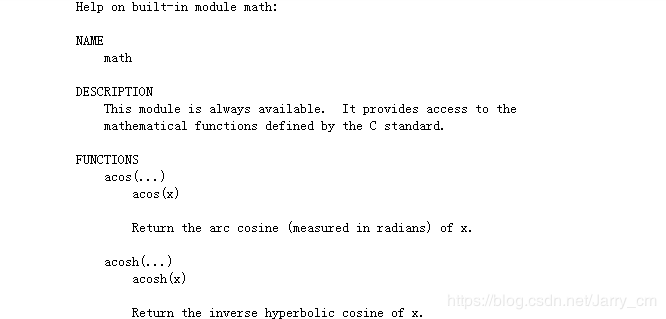## python内建函数在哪里

261次阅读python中内置了很多函数，这些函数实现了很多的功能。

```import math
dir(math)```

```['__doc__',
'__name__',
'__package__',
'__spec__',
'acos',
'acosh',
'asin',
'asinh',
'atan',
'atan2',
'atanh',
'ceil',
'copysign',
'cos',
'cosh',
'degrees',
'e',
'erf',
'erfc',
'exp',
'expm1',
'fabs',
'factorial',
'floor',
'fmod',
'frexp',
'fsum',
'gamma',
'gcd',
'hypot',
'inf',
'isclose',
'isfinite',
'isinf',
'isnan',
'ldexp',
'lgamma',
'log',
'log10',
'log1p',
'log2',
'modf',
'nan',
'pi',
'pow',
'sin',
'sinh',
'sqrt',
'tan',
'tanh',
'tau',
'trunc']```

```import math
help(math)``````import random
random.__file__```

`'D:\\Anaconda2\\envs\\py3\\lib\\random.py'`